# Pie charts#

Demo of plotting a pie chart.

This example illustrates various parameters of pie.

## Label slices#

Plot a pie chart of animals and label the slices. To add labels, pass a list of labels to the labels parameter

import matplotlib.pyplot as plt
labels = 'Frogs', 'Hogs', 'Dogs', 'Logs'
sizes = [15, 30, 45, 10]

fig, ax = plt.subplots()
ax.pie(sizes, labels=labels)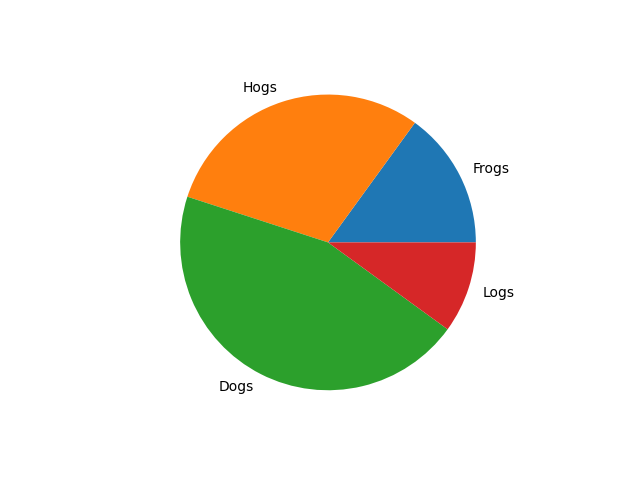Each slice of the pie chart is a patches.Wedge object; therefore in addition to the customizations shown here, each wedge can be customized using the wedgeprops argument, as demonstrated in Nested pie charts.

## Auto-label slices#

Pass a function or format string to autopct to label slices.

fig, ax = plt.subplots()
ax.pie(sizes, labels=labels, autopct='%1.1f%%')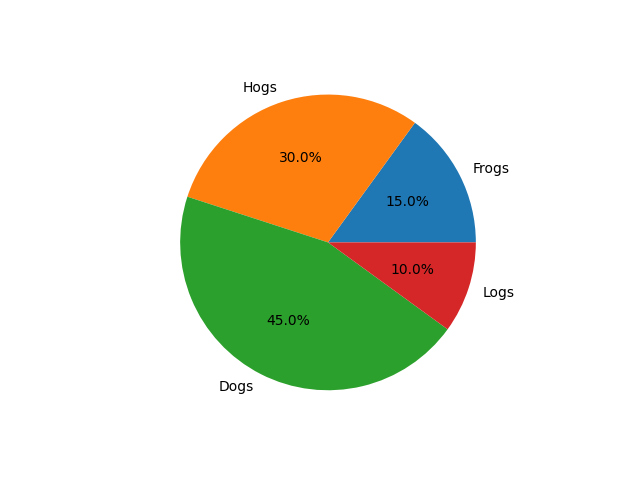By default, the label values are obtained from the percent size of the slice.

## Color slices#

Pass a list of colors to colors to set the color of each slice.

fig, ax = plt.subplots()
ax.pie(sizes, labels=labels,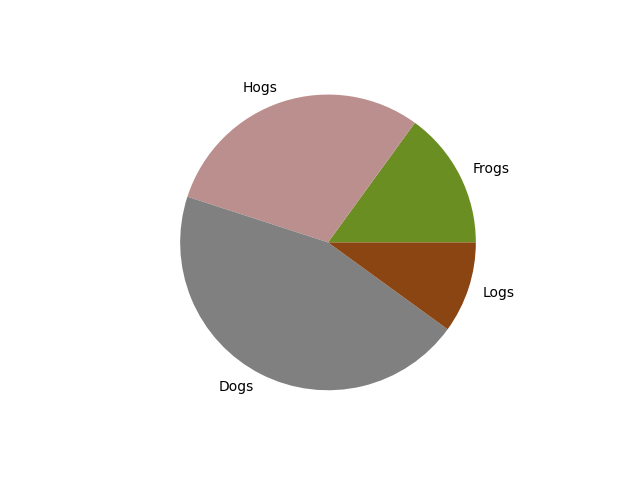## Hatch slices#

Pass a list of hatch patterns to hatch to set the pattern of each slice.

fig, ax = plt.subplots()
ax.pie(sizes, labels=labels, hatch=['**O', 'oO', 'O.O', '.||.'])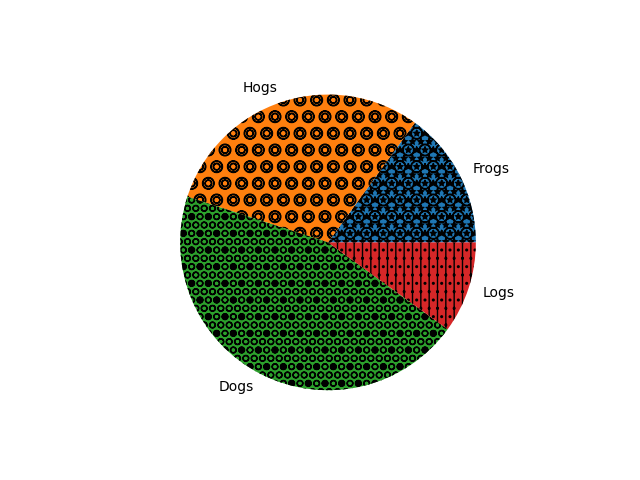## Swap label and autopct text positions#

Use the labeldistance and pctdistance parameters to position the labels and autopct text respectively.

fig, ax = plt.subplots()
ax.pie(sizes, labels=labels, autopct='%1.1f%%',
pctdistance=1.25, labeldistance=.6)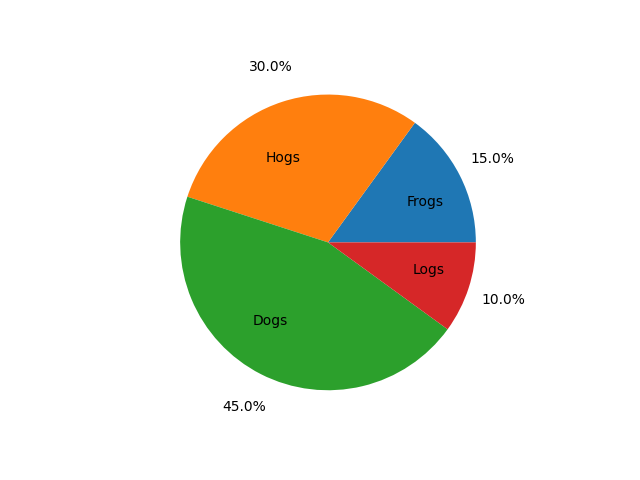labeldistance and pctdistance are ratios of the radius; therefore they vary between 0 for the center of the pie and 1 for the edge of the pie, and can be set to greater than 1 to place text outside the pie.

## Explode, shade, and rotate slices#

In addition to the basic pie chart, this demo shows a few optional features:

• offsetting a slice using explode

• custom start angle using startangle

This example orders the slices, separates (explodes) them, and rotates them.

explode = (0, 0.1, 0, 0)  # only "explode" the 2nd slice (i.e. 'Hogs')

fig, ax = plt.subplots()
ax.pie(sizes, explode=explode, labels=labels, autopct='%1.1f%%',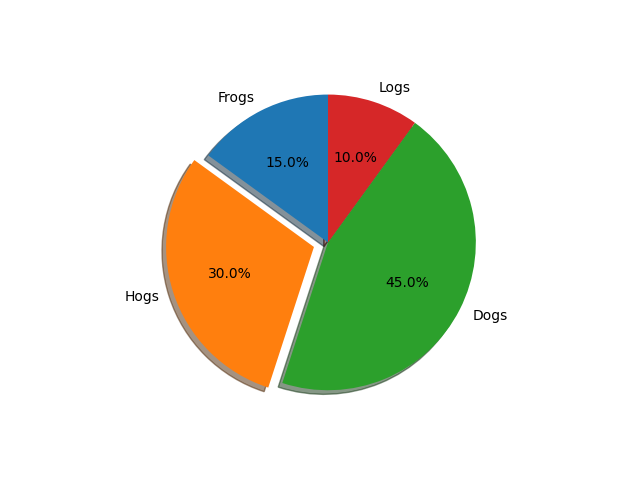The default startangle is 0, which would start the first slice ("Frogs") on the positive x-axis. This example sets startangle = 90 such that all the slices are rotated counter-clockwise by 90 degrees, and the frog slice starts on the positive y-axis.